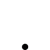# 麻煩小幫手，請問這樣寫哪裡出現問題了？

•   14
• 最後發表   Sukibayashi  2 週前
Sukibayashi 發文於   2021/06/10

value1=getfield("漲停價", "D");

value2=countif(close=value1,226);

if close>20 then ret = 1;

outputField1(value2,"漲停次數");

// 用選股策略列出的結果只會顯示當天是漲停板為1，不是漲停板為0

input:daymin(226,"查看範圍");

var:i(0),count(0);

value1=getfield("漲停價", "D");

for i=0 to daymin

begin

if close[i]=value1 then

begin

count+=1;

end;

end;

if close>20 then ret=1;

outputField1(count,"漲停次數");

// 用選股中心執行後，結果有誤。

XQ小幫手 發文於   2021/06/11

Hello Sukibayashi,

input:daymin(226,"查看範圍");

var:i(0),count(0);

count = 0;

for i=0 to daymin begin

if close[i] = getfield("漲停價")[i] then count+=1;

end;

Sukibayashi 發文於   2021/06/11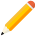發表新主題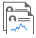我的發文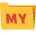我的收藏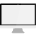分類

Close